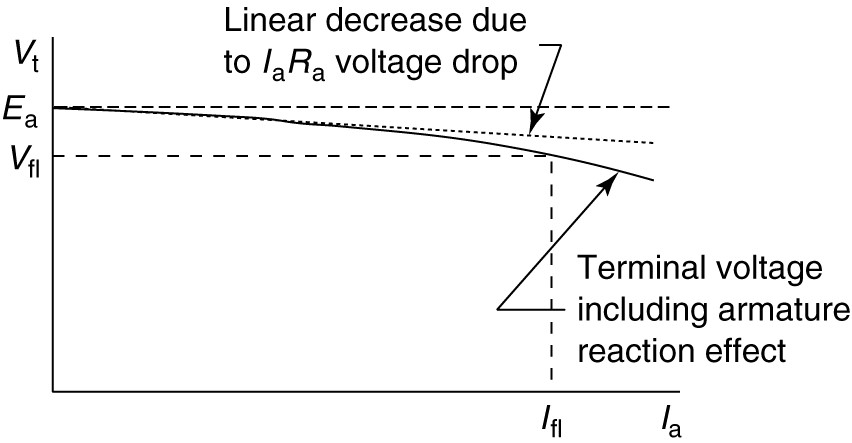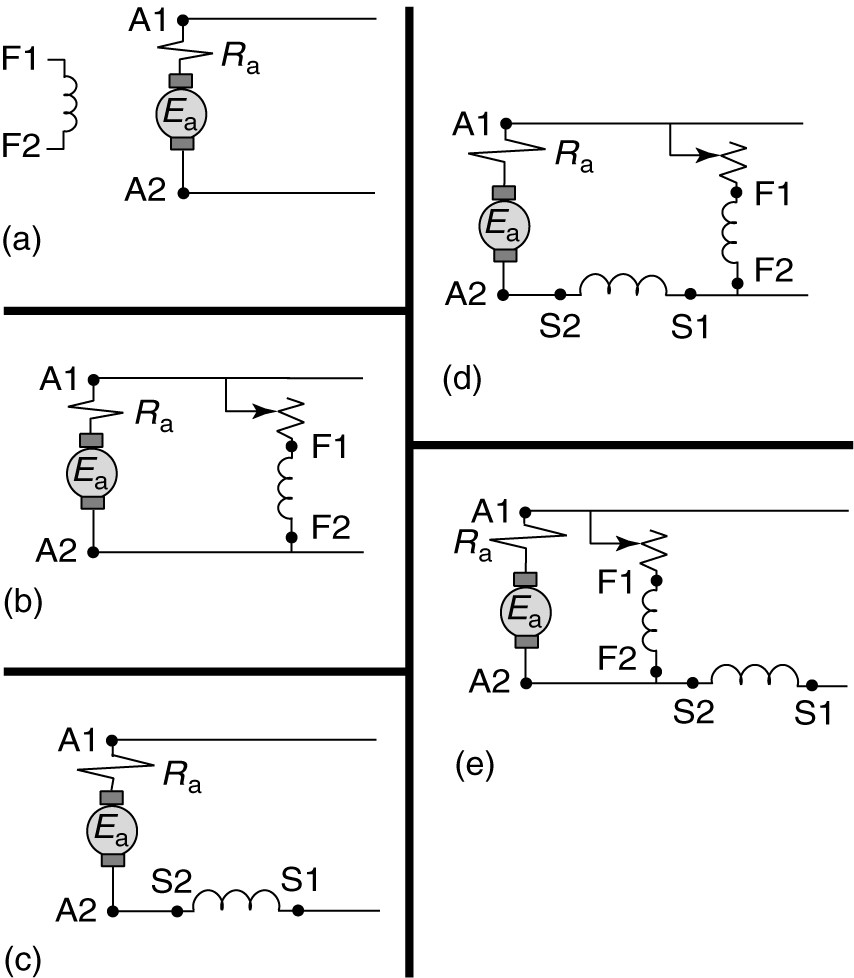Home / DC Machines / DC Generator Characteristics

# DC Generator Characteristics

Want create site? Find Free WordPress Themes and plugins.

As shown by equation 1, the terminal voltage of the separately excited DC generator would be expected to decrease in a linear manner due to the voltage drop across the armature circuit resistance.

$\begin{matrix} {{V}_{t}}={{E}_{a}}-{{I}_{a}}{{R}_{a}} & {} & \left( 1 \right) \\\end{matrix}$

Figure 1 shows the linear decrease that would be expected from equation 2. In fact, however, as the load current increases, armature reaction may lower the flux per pole, causing an additional reduction in the terminal voltage. Thus, the terminal voltage of a separately excited DC generator will actually decline nonlinearly when the armature current is increased, as shown by the solid line in Figure 1.

$\begin{matrix} VR=\frac{{{V}_{nl}}-{{V}_{fl}}}{{{V}_{fl}}}\times 100 & {} & \left( 2 \right) \\\end{matrix}$

In order to properly calculate the voltage regulation of the machine, we must account for the armature reaction.FIGURE 1:  Effect of armature reaction on separately excited generator terminal voltage.

The curves in Figure 1 started together at zero current and diverged as the current increased. Redrawing the voltage curves so they converge at the rated voltage, as shown in Figure 2, shows the effect of armature reaction and suggests a method to account for it.FIGURE 2:  Correcting for armature reaction.

Applying equation 1 to the rated terminal voltage and current, we could calculate the generated voltage, Ea, at full load, as shown in Figure 2. However, we know that the generated voltage will be higher at no load.

Essentially, armature reaction causes the generated voltage to change with the load current. Armature reaction is usually expressed as a reduction in the effective field current or MMF at full load. For example, armature reaction might have the effect of reducing the applied MMF by 6%, which means that the applied MMF is only 94% effective.

If we know the terminal voltage at full load, we can calculate the generated voltage Ea. Using a magnetization curve for the machine in question, we can find the effective MMF, or field current, required to generate this voltage. Then we can divide the MMF required at no load by 0.94 to find the actual MMF that must be present at full load to overcome the armature reaction. Using that value of MMF we can find the actual no-load voltage.

The field windings in a DC generator may be separately excited from a DC power supply, or the generator may be self-excited, which means the machine provides its own excitation. Figures 3 (b), (c), (d), and (e) showed the various types of field connections. Each type of field connection will result in different volt-ampere characteristics.FIGURE 3:    Field connections for the DC machine.  a. Separately excited.  b. Shunt-motor or self-excited generator.  c. Series machine.  d. Long-shunt, compound connection.  e. Short-shunt, compound connection.

Figure 4 shows volt-ampere characteristics for the different connections of DC generators. All of these curves are based on the machine being turned at a constant speed.

As we have described, the generated voltage of the separately excited machine will have a drop as the current increases from no load to full load, assuming the field current is held constant. This drop is due to the resistive drop across the armature winding and to the net reduction in flux that is caused by armature reaction.

The volt-amp characteristic of the separately excited generator is shown by the heavy solid line in Figure 4 and will serve as the base case against which to compare other types of generators.FIGURE 4:  Volt-amp characteristics of DC generators.

The self-excited generator, shown in Figure 3 (b), is similar to the separately excited generator; however, the voltage regulation is higher. The voltage applied to the field is the output of the generator, so as the load increases, the terminal voltage decreases, which means the field receives less voltage. Because less voltage is applied to the field, there is less field current and less flux is created. Less flux reduces the generated voltage, driving the terminal voltage down even more.

Thus, if a self- excited machine is to deliver rated voltage at rated current, the no-load voltage must be higher than if the machine were separately excited, as shown by the heavy dashed line in Figure 4.

The series DC generator uses the armature current as the field current. Therefore, the field winding produces no magnetic flux at no load, so the only flux available to generate a voltage is due to any residual magnetism in the iron of the stator.

The residual magnetism is sufficient to produce only a few volts at no load. As the load current increases, the flux, and thus the generated voltage, increases.

Eventually, of course, saturation effects will cause the voltage to level off. As a result, the volt-amp characteristic of the series DC generator looks like a magnetization curve, as shown by the dashed-and-dotted line of Figure 4.

Compound generators, as shown in Figures 3(d) and (e), contain both a series and a shunt winding. The characteristics of a compound generator are a function of the relative strengths of the two field windings and whether they are cumulatively or differentially connected.

Cumulatively connected means the two field windings produce flux in the same direction. The circuits as labeled in Figure 3 are cumulatively connected.

A differential connection means that the series winding produces a flux that opposes the flux produced by the shunt winding.

The voltage regulation of a cumulatively compounded DC generator can be positive, negative, or even zero, depending on the flux produced by the two field windings.

Figure 4 shows a volt-amp characteristic for which the voltage regulation is zero percent; i.e., the voltage is the same at no load and full load. This is a so-called flat compounded machine.

Did you find apk for android? You can find new Free Android Games and apps.

### About Ahmad FaizanMr. Ahmed Faizan Sheikh, M.Sc. (USA), Research Fellow (USA), a member of IEEE & CIGRE, is a Fulbright Alumnus and earned his Master’s Degree in Electrical and Power Engineering from Kansas State University, USA.